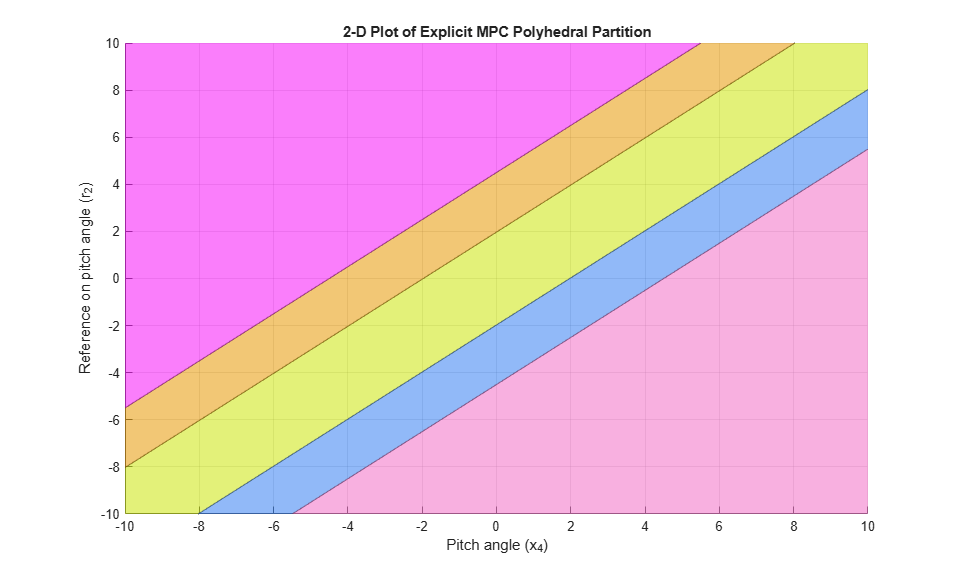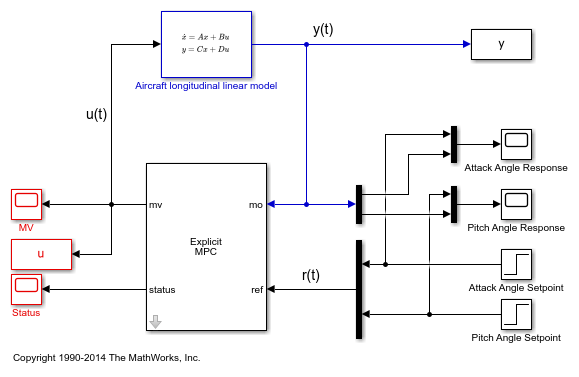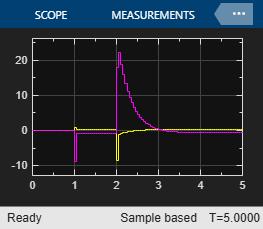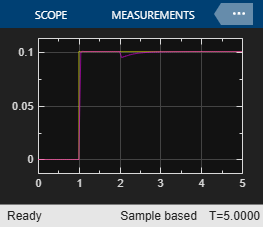# Explicit MPC Control of an Aircraft with Unstable Poles

This example shows how to control an unstable aircraft with saturating actuators using explicit model predictive control.

For an example that controls the same plant using a traditional MPC controller, see MPC Control of an Aircraft with Unstable Poles.

### Define Aircraft Model

The linear open-loop dynamic model of the aircraft has the following state-space matrices:

```A = [-0.0151 -60.5651 0 -32.174; -0.0001 -1.3411 0.9929 0; 0.00018 43.2541 -0.86939 0; 0 0 1 0]; B = [-2.516 -13.136; -0.1689 -0.2514; -17.251 -1.5766; 0 0]; C = [0 1 0 0; 0 0 0 1]; D = [0 0; 0 0]; ```

Create the plant, and specify the initial states as zero.

```plant = ss(A,B,C,D); x0 = zeros(4,1); ```

The manipulated variables are the elevator and flaperon angles. The attack and pitch angles are measured outputs to be regulated.

The open-loop response of the system is unstable.

```pole(plant) ```
```ans = -7.6636 + 0.0000i 5.4530 + 0.0000i -0.0075 + 0.0556i -0.0075 - 0.0556i ```

### Design MPC Controller

To obtain an Explicit MPC controller, you must first design a traditional (implicit) model predictive controller that is able to achieve your control objectives.

MV Constraints

Both manipulated variables are constrained between +/- 25 degrees. Since the plant inputs and outputs are of different orders of magnitude, you also use scale factors to facilitate MPC tuning. Typical choices of scale factor are the upper/lower limit or the operating range.

```MV = struct('Min',{-25,-25},'Max',{25,25},'ScaleFactor',{50,50}); ```

OV Constraints

Both plant outputs have constraints to limit undershoot at the first prediction horizon step. Also, specify scale factors for outputs.

```OV = struct('Min',{[-0.5;-Inf],[-100;-Inf]},'Max',{[0.5;Inf],[100;Inf]},'ScaleFactor',{1,200}); ```

Weights

The control task is to get zero offset for piecewise-constant references, while avoiding instability due to input saturation. Because both MV and OV variables are already scaled in MPC controller, MPC weights are dimensionless and applied to the scaled MV and OV values. In this example, you penalize the two outputs equally with the same OV weights.

```Weights = struct('MV',[0 0],'MVRate',[0.1 0.1],'OV',[10 10]); ```

Create an MPC controller with the specified plant model, sample time, and horizons.

```Ts = 0.05; % Sample time p = 10; % Prediction horizon m = 2; % Control horizon mpcobj = mpc(plant,Ts,p,m,Weights,MV,OV); ```

### Generate Explicit MPC Controller

Explicit MPC executes the equivalent explicit piecewise affine version of the MPC control law defined by the traditional MPC controller. To generate an explicit MPC controller from a traditional MPC controller, you must specify the range for each controller state, reference signal, manipulated variable and measured disturbance. Doing so ensures that the multi-parametric quadratic programming problem is solved in the parameter space defined by these ranges.

Obtain a range structure for initialization

To obtain a range structure where you can specify the range for each parameter, use the `generateExplicitRange` command.

```range = generateExplicitRange(mpcobj); ```
```-->Converting model to discrete time. -->Assuming output disturbance added to measured output channel #1 is integrated white noise. -->Assuming output disturbance added to measured output channel #2 is integrated white noise. -->The "Model.Noise" property of the "mpc" object is empty. Assuming white noise on each measured output channel. ```

Specify Ranges for Controller States

MPC controller states include states from the plant model, disturbance model, and noise model, in that order. Setting the range of a state variable is sometimes difficult when the state does not correspond to a physical parameter. In that case, multiple runs of open-loop plant simulation with typical reference and disturbance signals are recommended in order to collect data that reflect the ranges of states.

```range.State.Min(:) = -10000; range.State.Max(:) = 10000; ```

Specify Ranges for Reference Signals

Usually you know the practical range of the reference signals being used at the nominal operating point in the plant. The ranges used to generate an explicit MPC controller must be at least as large as the practical range.

```range.Reference.Min = [-1;-11]; range.Reference.Max = [1;11]; ```

Specify Ranges for Manipulated Variables

If manipulated variables are constrained, the ranges used to generate an explicit MPC controller must be at least as large as these limits.

```range.ManipulatedVariable.Min = [MV(1).Min; MV(2).Min] - 1; range.ManipulatedVariable.Max = [MV(1).Max; MV(2).Max] + 1; ```

Construct Explicit MPC Controller

Use `generateExplicitMPC` command to obtain the explicit MPC controller with the parameter ranges previously specified.

```mpcobjExplicit = generateExplicitMPC(mpcobj, range); display(mpcobjExplicit) ```
``` Regions found / unexplored: 483/ 0 Explicit MPC Controller --------------------------------------------- Controller sample time: 0.05 (seconds) Polyhedral regions: 483 Number of parameters: 10 Is solution simplified: No State Estimation: Default Kalman gain --------------------------------------------- Type 'mpcobjExplicit.MPC' for the original implicit MPC design. Type 'mpcobjExplicit.Range' for the valid range of parameters. Type 'mpcobjExplicit.OptimizationOptions' for the options used in multi-parametric QP computation. Type 'mpcobjExplicit.PiecewiseAffineSolution' for regions and gain in each solution. ```

To join pairs of regions whose corresponding gains are the same and whose union is a convex set, use the `simplify` command with the `'exact'` method. This practice can reduce the memory footprint of the explicit MPC controller without sacrificing performance.

```mpcobjExplicitSimplified = simplify(mpcobjExplicit, 'exact'); display(mpcobjExplicitSimplified) ```
``` Regions to analyze: 471/ 471 Explicit MPC Controller --------------------------------------------- Controller sample time: 0.05 (seconds) Polyhedral regions: 471 Number of parameters: 10 Is solution simplified: Yes State Estimation: Default Kalman gain --------------------------------------------- Type 'mpcobjExplicitSimplified.MPC' for the original implicit MPC design. Type 'mpcobjExplicitSimplified.Range' for the valid range of parameters. Type 'mpcobjExplicitSimplified.OptimizationOptions' for the options used in multi-parametric QP computation. Type 'mpcobjExplicitSimplified.PiecewiseAffineSolution' for regions and gain in each solution. ```

The number of piecewise affine regions has been reduced.

### Plot Piecewise Affine Partition

You can review any 2-D section of the piecewise affine partition defined by the Explicit MPC control law.

Obtain a plot parameter structure for initialization

To obtain a parameter structure where you can specify which 2-D section to plot, use the `generatePlotParameters` function.

```params = generatePlotParameters(mpcobjExplicitSimplified); ```

Specify parameters for a 2-D plot

In this example, you plot the pitch angle (the 4th state variable) vs. its reference (the 2nd reference signal). All the other parameters must be fixed at values within their respective ranges.

Fix other state variables.

```params.State.Index = [1 2 3 5 6]; params.State.Value = [0 0 0 0 0]; ```

Fix other reference signals.

```params.Reference.Index = 1; params.Reference.Value = 0; ```

Fix manipulated variables.

```params.ManipulatedVariable.Index = [1 2]; params.ManipulatedVariable.Value = [0 0]; ```

Plot the 2-D section

Use `plotSection` command to plot the 2-D section defined previously.

```plotSection(mpcobjExplicitSimplified,params); axis([-10 10 -10 10]) grid xlabel('Pitch angle (x_4)') ylabel('Reference on pitch angle (r_2)') ```To run this example, Simulink® is required.

```if ~mpcchecktoolboxinstalled('simulink') disp('Simulink(R) is required to run this example.') return end ```

Simulate closed-loop control of the linear plant model in Simulink. To do so, for the MPC Controller block, set the Explicit MPC Controller property to `mpcobjExplicitSimplified`.

```mdl = 'empc_aircraft'; open_system(mdl) sim(mdl) ```The closed-loop response is identical to the traditional MPC controller designed in MPC Control of an Aircraft with Unstable Poles.

### References

 P. Kapasouris, M. Athans, and G. Stein, "Design of feedback control systems for unstable plants with saturating actuators", Proc. IFAC Symp. on Nonlinear Control System Design, Pergamon Press, pp.302--307, 1990

 A. Bemporad, A. Casavola, and E. Mosca, "Nonlinear control of constrained linear systems via predictive reference management", IEEE® Trans. Automatic Control, vol. AC-42, no. 3, pp. 340-349, 1997.

```bdclose(mdl) ```